# Mean State

Period Mean (original grids) [W/m2]
Model Period Mean (intersection) [W/m2]
Model Period Mean (complement) [W/m2]
Benchmark Period Mean (intersection) [W/m2]
Benchmark Period Mean (complement) [W/m2]
Bias [W/m2]
RMSE [W/m2]
Phase Shift [months]
Bias Score 
RMSE Score 
Seasonal Cycle Score 
Spatial Distribution Score 
Interannual Variability Score 
Overall Score 
Benchmark [-] 131.
CRUNCEPv7 [-] 136. 136. 0.00 130. 157. 6.69 21.4 1.30 0.46 0.45 0.83 0.98 0.53 0.62
GSWP3v1 [-] 116. 116. 0.00 130. 157. -13.7 22.6 1.40 0.51 0.42 0.81 0.99 0.69 0.64
WATCH [-] 84.6 84.3 0.00 129. 156. -44.0 48.9 2.20 0.14 0.36 0.65 0.88 0.69 0.51
Period Mean (original grids) [W/m2]
Model Period Mean (intersection) [W/m2]
Model Period Mean (complement) [W/m2]
Benchmark Period Mean (intersection) [W/m2]
Benchmark Period Mean (complement) [W/m2]
Bias [W/m2]
RMSE [W/m2]
Phase Shift [months]
Bias Score 
RMSE Score 
Seasonal Cycle Score 
Spatial Distribution Score 
Interannual Variability Score 
Overall Score 
Benchmark [-] 123.
CRUNCEPv7 [-] 109. 108. 0.00 122. 160. -13.3 25.3 1.52 0.55 0.47 0.77 0.99 0.63 0.65
GSWP3v1 [-] 97.4 96.9 0.00 122. 160. -24.8 30.3 1.43 0.37 0.50 0.79 0.94 0.65 0.63
WATCH [-] 77.3 77.3 0.00 121. 157. -43.2 46.7 1.50 0.21 0.46 0.78 0.76 0.71 0.56
Period Mean (original grids) [W/m2]
Model Period Mean (intersection) [W/m2]
Model Period Mean (complement) [W/m2]
Benchmark Period Mean (intersection) [W/m2]
Benchmark Period Mean (complement) [W/m2]
Bias [W/m2]
RMSE [W/m2]
Phase Shift [months]
Bias Score 
RMSE Score 
Seasonal Cycle Score 
Spatial Distribution Score 
Interannual Variability Score 
Overall Score 
Benchmark [-] 123.
CRUNCEPv7 [-] 114. 114. 0.00 121. 157. -5.34 20.3 1.24 0.70 0.61 0.84 1.0 0.57 0.72
GSWP3v1 [-] 105. 105. 0.00 121. 157. -15.0 25.1 1.49 0.62 0.57 0.77 0.96 0.68 0.69
WATCH [-] 99.8 99.7 0.00 119. 151. -18.2 31.6 2.10 0.51 0.51 0.67 0.67 0.73 0.60
Period Mean (original grids) [W/m2]
Model Period Mean (intersection) [W/m2]
Model Period Mean (complement) [W/m2]
Benchmark Period Mean (intersection) [W/m2]
Benchmark Period Mean (complement) [W/m2]
Bias [W/m2]
RMSE [W/m2]
Phase Shift [months]
Bias Score 
RMSE Score 
Seasonal Cycle Score 
Spatial Distribution Score 
Interannual Variability Score 
Overall Score 
Benchmark [-] 34.4
CRUNCEPv7 [-] 15.2 15.2 0.00 34.5 33.0 -20.7 27.5 0.421 0.70 0.73 0.97 0.78 0.66 0.76
GSWP3v1 [-] 32.9 33.0 0.00 34.5 33.0 -3.11 20.8 0.266 0.86 0.72 0.98 0.82 0.74 0.81
WATCH [-] 22.0 22.0 0.00 39.6 37.2 -17.4 22.7 0.382 0.73 0.74 0.97 0.97 0.71 0.81
Period Mean (original grids) [W/m2]
Model Period Mean (intersection) [W/m2]
Model Period Mean (complement) [W/m2]
Benchmark Period Mean (intersection) [W/m2]
Benchmark Period Mean (complement) [W/m2]
Bias [W/m2]
RMSE [W/m2]
Phase Shift [months]
Bias Score 
RMSE Score 
Seasonal Cycle Score 
Spatial Distribution Score 
Interannual Variability Score 
Overall Score 
Benchmark [-] 147.
CRUNCEPv7 [-] 157. 157. 0.00 145. 156. 14.9 23.6 1.65 0.42 0.44 0.76 1.0 0.51 0.59
GSWP3v1 [-] 128. 127. 0.00 145. 156. -13.0 21.7 1.63 0.48 0.43 0.74 0.99 0.69 0.63
WATCH [-] 100. 100. 0.00 145. 152. -37.4 42.6 2.53 0.19 0.33 0.58 0.57 0.59 0.43
Period Mean (original grids) [W/m2]
Model Period Mean (intersection) [W/m2]
Model Period Mean (complement) [W/m2]
Benchmark Period Mean (intersection) [W/m2]
Benchmark Period Mean (complement) [W/m2]
Bias [W/m2]
RMSE [W/m2]
Phase Shift [months]
Bias Score 
RMSE Score 
Seasonal Cycle Score 
Spatial Distribution Score 
Interannual Variability Score 
Overall Score 
Benchmark [-] 53.7
CRUNCEPv7 [-] 43.0 42.9 0.00 53.5 64.9 -10.5 22.6 0.391 0.83 0.72 0.97 0.86 0.78 0.81
GSWP3v1 [-] 48.1 48.1 0.00 53.5 64.9 -5.14 17.0 0.214 0.87 0.77 0.99 0.90 0.72 0.84
WATCH [-] 39.6 39.6 0.00 59.1 68.9 -19.2 20.5 0.228 0.72 0.78 0.98 0.99 0.74 0.83
Period Mean (original grids) [W/m2]
Model Period Mean (intersection) [W/m2]
Model Period Mean (complement) [W/m2]
Benchmark Period Mean (intersection) [W/m2]
Benchmark Period Mean (complement) [W/m2]
Bias [W/m2]
RMSE [W/m2]
Phase Shift [months]
Bias Score 
RMSE Score 
Seasonal Cycle Score 
Spatial Distribution Score 
Interannual Variability Score 
Overall Score 
Benchmark [-] 71.7
CRUNCEPv7 [-] 66.2 66.0 0.00 71.1 90.6 -3.81 21.4 0.517 0.79 0.69 0.95 0.94 0.73 0.80
GSWP3v1 [-] 62.6 62.6 0.00 71.1 90.6 -7.26 19.0 0.429 0.81 0.72 0.96 0.99 0.71 0.82
WATCH [-] 57.2 56.9 0.00 77.3 97.4 -19.2 24.2 0.697 0.66 0.68 0.94 0.98 0.70 0.77
Period Mean (original grids) [W/m2]
Model Period Mean (intersection) [W/m2]
Model Period Mean (complement) [W/m2]
Benchmark Period Mean (intersection) [W/m2]
Benchmark Period Mean (complement) [W/m2]
Bias [W/m2]
RMSE [W/m2]
Phase Shift [months]
Bias Score 
RMSE Score 
Seasonal Cycle Score 
Spatial Distribution Score 
Interannual Variability Score 
Overall Score 
Benchmark [-] 120.
CRUNCEPv7 [-] 121. 121. 0.00 118. 153. 7.47 23.1 0.885 0.65 0.57 0.90 0.96 0.62 0.71
GSWP3v1 [-] 95.0 94.6 0.00 118. 153. -18.8 27.5 0.690 0.55 0.54 0.92 0.99 0.65 0.70
WATCH [-] 82.3 82.4 0.00 120. 153. -33.0 38.6 0.806 0.38 0.51 0.90 0.98 0.71 0.67
Period Mean (original grids) [W/m2]
Model Period Mean (intersection) [W/m2]
Model Period Mean (complement) [W/m2]
Benchmark Period Mean (intersection) [W/m2]
Benchmark Period Mean (complement) [W/m2]
Bias [W/m2]
RMSE [W/m2]
Phase Shift [months]
Bias Score 
RMSE Score 
Seasonal Cycle Score 
Spatial Distribution Score 
Interannual Variability Score 
Overall Score 
Benchmark [-] 30.5
CRUNCEPv7 [-] 10.3 10.7 0.00 31.0 19.5 -21.6 27.9 0.400 0.68 0.72 0.97 0.85 0.71 0.78
GSWP3v1 [-] 24.2 24.5 0.00 31.0 19.5 -7.63 19.3 0.205 0.86 0.74 0.99 0.98 0.75 0.84
WATCH [-] 15.9 16.1 0.00 36.4 25.1 -20.8 24.7 0.138 0.69 0.75 0.99 0.82 0.70 0.78
Period Mean (original grids) [W/m2]
Model Period Mean (intersection) [W/m2]
Model Period Mean (complement) [W/m2]
Benchmark Period Mean (intersection) [W/m2]
Benchmark Period Mean (complement) [W/m2]
Bias [W/m2]
RMSE [W/m2]
Phase Shift [months]
Bias Score 
RMSE Score 
Seasonal Cycle Score 
Spatial Distribution Score 
Interannual Variability Score 
Overall Score 
Benchmark [-] 109.
CRUNCEPv7 [-] 98.8 98.7 0.00 108. 131. -7.39 17.6 0.707 0.82 0.73 0.94 0.98 0.68 0.81
GSWP3v1 [-] 93.6 93.6 0.00 108. 131. -12.2 20.1 0.514 0.75 0.73 0.96 0.96 0.77 0.82
WATCH [-] 104. 104. 0.00 108. 124. -3.32 20.5 0.842 0.82 0.66 0.91 0.87 0.70 0.77
Period Mean (original grids) [W/m2]
Model Period Mean (intersection) [W/m2]
Model Period Mean (complement) [W/m2]
Benchmark Period Mean (intersection) [W/m2]
Benchmark Period Mean (complement) [W/m2]
Bias [W/m2]
RMSE [W/m2]
Phase Shift [months]
Bias Score 
RMSE Score 
Seasonal Cycle Score 
Spatial Distribution Score 
Interannual Variability Score 
Overall Score 
Benchmark [-] 65.7
CRUNCEPv7 [-] 62.1 62.2 0.00 63.8 85.4 -0.888 22.1 0.271 0.86 0.72 0.98 0.96 0.80 0.84
GSWP3v1 [-] 54.7 54.7 0.00 63.8 85.4 -6.96 17.5 0.197 0.87 0.78 0.99 0.99 0.68 0.85
WATCH [-] 40.5 40.5 0.00 67.7 91.0 -25.1 26.5 0.548 0.66 0.77 0.96 0.86 0.67 0.78
Period Mean (original grids) [W/m2]
Model Period Mean (intersection) [W/m2]
Model Period Mean (complement) [W/m2]
Benchmark Period Mean (intersection) [W/m2]
Benchmark Period Mean (complement) [W/m2]
Bias [W/m2]
RMSE [W/m2]
Phase Shift [months]
Bias Score 
RMSE Score 
Seasonal Cycle Score 
Spatial Distribution Score 
Interannual Variability Score 
Overall Score 
Benchmark [-] 109.
CRUNCEPv7 [-] 72.0 72.0 0.00 80.5 123. -6.85 22.6 0.734 0.66 0.62 0.92 0.96 0.62 0.73
GSWP3v1 [-] 65.1 65.1 0.00 80.5 123. -13.7 23.0 0.692 0.64 0.61 0.92 0.98 0.68 0.74
WATCH [-] 58.2 58.2 0.00 82.1 122. -22.3 28.7 1.06 0.53 0.60 0.86 0.97 0.69 0.71
Period Mean (original grids) [W/m2]
Model Period Mean (intersection) [W/m2]
Model Period Mean (complement) [W/m2]
Benchmark Period Mean (intersection) [W/m2]
Benchmark Period Mean (complement) [W/m2]
Bias [W/m2]
RMSE [W/m2]
Phase Shift [months]
Bias Score 
RMSE Score 
Seasonal Cycle Score 
Spatial Distribution Score 
Interannual Variability Score 
Overall Score 
Benchmark [-] 76.6
CRUNCEPv7 [-] 64.6 64.6 0.00 73.9 144. -7.02 19.7 0.916 0.68 0.62 0.90 0.95 0.62 0.73
GSWP3v1 [-] 53.6 53.6 0.00 73.9 144. -18.0 24.8 0.888 0.56 0.62 0.90 0.94 0.71 0.72
WATCH [-] 40.4 40.5 0.00 76.4 148. -33.9 34.9 1.21 0.31 0.61 0.85 0.84 0.71 0.65
Period Mean (original grids) [W/m2]
Model Period Mean (intersection) [W/m2]
Model Period Mean (complement) [W/m2]
Benchmark Period Mean (intersection) [W/m2]
Benchmark Period Mean (complement) [W/m2]
Bias [W/m2]
RMSE [W/m2]
Phase Shift [months]
Bias Score 
RMSE Score 
Seasonal Cycle Score 
Spatial Distribution Score 
Interannual Variability Score 
Overall Score 
Benchmark [-] 36.2
CRUNCEPv7 [-] 15.1 15.0 0.00 36.2 36.3 -23.1 30.0 0.138 0.66 0.71 0.99 0.80 0.59 0.74
GSWP3v1 [-] 27.4 27.3 0.00 36.2 36.3 -10.9 21.5 0.0841 0.82 0.73 0.99 0.93 0.77 0.83
WATCH [-] 22.9 22.7 0.00 40.3 41.6 -18.3 24.0 0.201 0.72 0.72 0.99 0.95 0.71 0.80
Period Mean (original grids) [W/m2]
Model Period Mean (intersection) [W/m2]
Model Period Mean (complement) [W/m2]
Benchmark Period Mean (intersection) [W/m2]
Benchmark Period Mean (complement) [W/m2]
Bias [W/m2]
RMSE [W/m2]
Phase Shift [months]
Bias Score 
RMSE Score 
Seasonal Cycle Score 
Spatial Distribution Score 
Interannual Variability Score 
Overall Score 
Benchmark [-] 138.
CRUNCEPv7 [-] 116. 117. 0.00 133. 161. -8.35 28.0 1.05 0.53 0.63 0.87 0.80 0.68 0.69
GSWP3v1 [-] 107. 107. 0.00 133. 161. -20.2 27.5 1.29 0.56 0.62 0.83 0.99 0.70 0.72
WATCH [-] 98.5 98.6 0.00 133. 163. -29.8 33.3 1.48 0.42 0.59 0.80 0.90 0.71 0.67
Period Mean (original grids) [W/m2]
Model Period Mean (intersection) [W/m2]
Model Period Mean (complement) [W/m2]
Benchmark Period Mean (intersection) [W/m2]
Benchmark Period Mean (complement) [W/m2]
Bias [W/m2]
RMSE [W/m2]
Phase Shift [months]
Bias Score 
RMSE Score 
Seasonal Cycle Score 
Spatial Distribution Score 
Interannual Variability Score 
Overall Score 
Benchmark [-] 98.6
CRUNCEPv7 [-] 88.6 88.9 0.00 96.9 135. -6.07 19.5 0.395 0.86 0.73 0.97 0.97 0.66 0.82
GSWP3v1 [-] 81.8 81.9 0.00 96.9 135. -13.3 19.7 0.468 0.78 0.76 0.96 0.93 0.73 0.82
WATCH [-] 83.3 83.4 0.00 100. 141. -15.6 19.8 0.583 0.75 0.73 0.95 0.91 0.70 0.79
Period Mean (original grids) [W/m2]
Model Period Mean (intersection) [W/m2]
Model Period Mean (complement) [W/m2]
Benchmark Period Mean (intersection) [W/m2]
Benchmark Period Mean (complement) [W/m2]
Bias [W/m2]
RMSE [W/m2]
Phase Shift [months]
Bias Score 
RMSE Score 
Seasonal Cycle Score 
Spatial Distribution Score 
Interannual Variability Score 
Overall Score 
Benchmark [-] 63.9
CRUNCEPv7 [-] 54.7 54.8 0.00 63.6 72.9 -8.84 22.7 0.402 0.85 0.71 0.97 0.94 0.59 0.80
GSWP3v1 [-] 58.2 58.3 0.00 63.6 72.9 -5.21 15.3 0.153 0.90 0.79 0.99 0.99 0.71 0.86
WATCH [-] 55.1 55.3 0.00 67.8 79.0 -12.0 18.2 0.397 0.81 0.75 0.97 0.99 0.74 0.84
Period Mean (original grids) [W/m2]
Model Period Mean (intersection) [W/m2]
Model Period Mean (complement) [W/m2]
Benchmark Period Mean (intersection) [W/m2]
Benchmark Period Mean (complement) [W/m2]
Bias [W/m2]
RMSE [W/m2]
Phase Shift [months]
Bias Score 
RMSE Score 
Seasonal Cycle Score 
Spatial Distribution Score 
Interannual Variability Score 
Overall Score 
Benchmark [-] 82.3
CRUNCEPv7 [-] 63.0 63.5 0.00 81.8 110. -17.7 21.8 0.398 0.72 0.78 0.97 0.99 0.66 0.82
GSWP3v1 [-] 71.4 71.6 0.00 81.8 110. -9.78 18.5 0.542 0.81 0.77 0.95 0.95 0.74 0.83
WATCH [-] 71.3 71.7 0.00 87.4 114. -15.0 19.6 0.433 0.75 0.75 0.97 0.98 0.76 0.82
Period Mean (original grids) [W/m2]
Model Period Mean (intersection) [W/m2]
Model Period Mean (complement) [W/m2]
Benchmark Period Mean (intersection) [W/m2]
Benchmark Period Mean (complement) [W/m2]
Bias [W/m2]
RMSE [W/m2]
Phase Shift [months]
Bias Score 
RMSE Score 
Seasonal Cycle Score 
Spatial Distribution Score 
Interannual Variability Score 
Overall Score 
Benchmark [-] 139.
CRUNCEPv7 [-] 152. 152. 0.00 138. 170. 14.5 21.2 1.22 0.40 0.44 0.83 0.96 0.59 0.61
GSWP3v1 [-] 121. 122. 0.00 138. 170. -15.8 24.0 1.23 0.36 0.40 0.82 0.97 0.72 0.61
WATCH [-] 114. 114. 0.00 135. 169. -21.5 30.5 2.15 0.28 0.34 0.68 0.98 0.61 0.54
Period Mean (original grids) [W/m2]
Model Period Mean (intersection) [W/m2]
Model Period Mean (complement) [W/m2]
Benchmark Period Mean (intersection) [W/m2]
Benchmark Period Mean (complement) [W/m2]
Bias [W/m2]
RMSE [W/m2]
Phase Shift [months]
Bias Score 
RMSE Score 
Seasonal Cycle Score 
Spatial Distribution Score 
Interannual Variability Score 
Overall Score 
Benchmark [-] 121.
CRUNCEPv7 [-] 123. 122. 0.00 120. 143. 2.78 18.2 0.925 0.75 0.65 0.90 0.99 0.63 0.76
GSWP3v1 [-] 107. 107. 0.00 120. 143. -12.8 22.6 0.892 0.66 0.61 0.90 0.82 0.70 0.72
WATCH [-] 103. 103. 0.00 115. 138. -11.9 24.4 1.79 0.66 0.58 0.74 0.82 0.75 0.69
Period Mean (original grids) [W/m2]
Model Period Mean (intersection) [W/m2]
Model Period Mean (complement) [W/m2]
Benchmark Period Mean (intersection) [W/m2]
Benchmark Period Mean (complement) [W/m2]
Bias [W/m2]
RMSE [W/m2]
Phase Shift [months]
Bias Score 
RMSE Score 
Seasonal Cycle Score 
Spatial Distribution Score 
Interannual Variability Score 
Overall Score 
Benchmark [-] 98.1
CRUNCEPv7 [-] 83.8 83.4 0.00 95.8 118. -12.4 26.6 0.671 0.71 0.69 0.93 0.91 0.65 0.76
GSWP3v1 [-] 90.4 89.8 0.00 95.8 118. -7.08 23.4 0.560 0.76 0.71 0.95 0.99 0.64 0.79
WATCH [-] 87.7 87.4 0.00 91.4 111. -4.79 25.9 0.726 0.71 0.68 0.92 0.95 0.69 0.77

# Temporally integrated period mean

BENCHMARK MEAN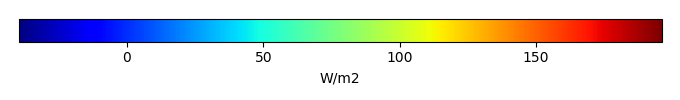MODEL MEANBIAS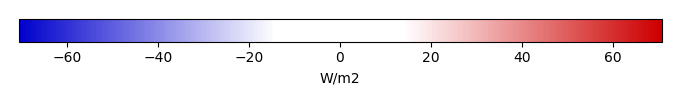BIAS SCORERMSE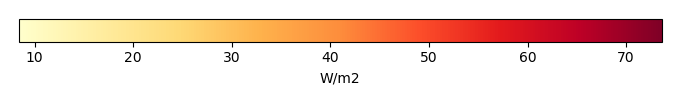RMSE SCOREBENCHMARK INTERANNUAL VARIABILITY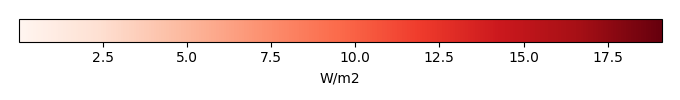MODEL INTERANNUAL VARIABILITYINTERANNUAL VARIABILITY SCOREBENCHMARK MAX MONTHMODEL MAX MONTHDIFFERENCE IN MAX MONTH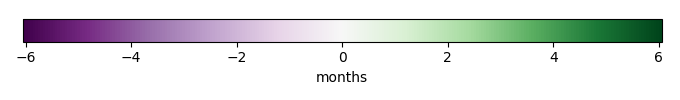SEASONAL CYCLE SCORESPATIAL TAYLOR DIAGRAMMODEL COLORS# Spatially integrated regional mean

MODEL COLORSREGIONAL MEANANNUAL CYCLEMONTHLY ANOMALYANNUAL CYCLE# All Models

BenchmarkCRUNCEPv7GSWP3v1WATCH# Data Information

creation_date: Thu May 8 22:58:12 PDT 2014

source_file: This product is generated from monthly 1 degree CERES EBAF Radiation observations